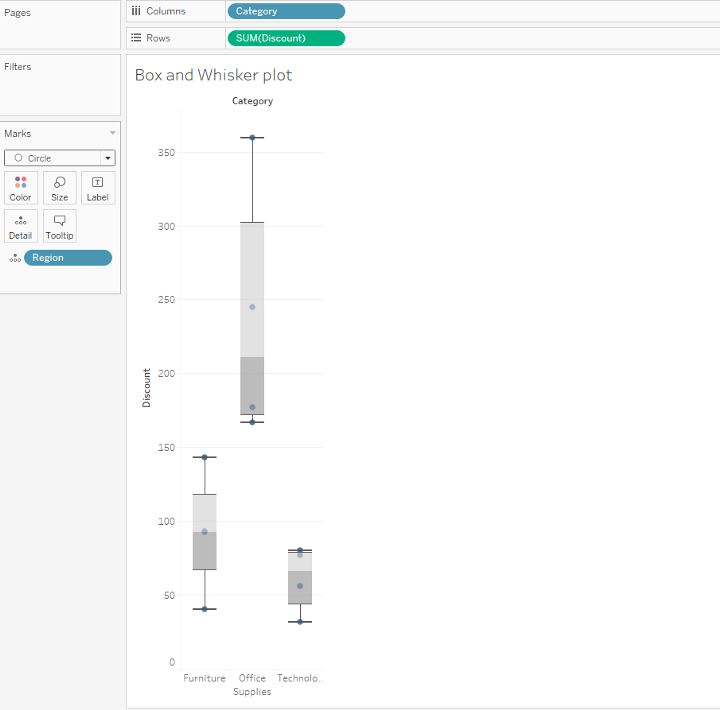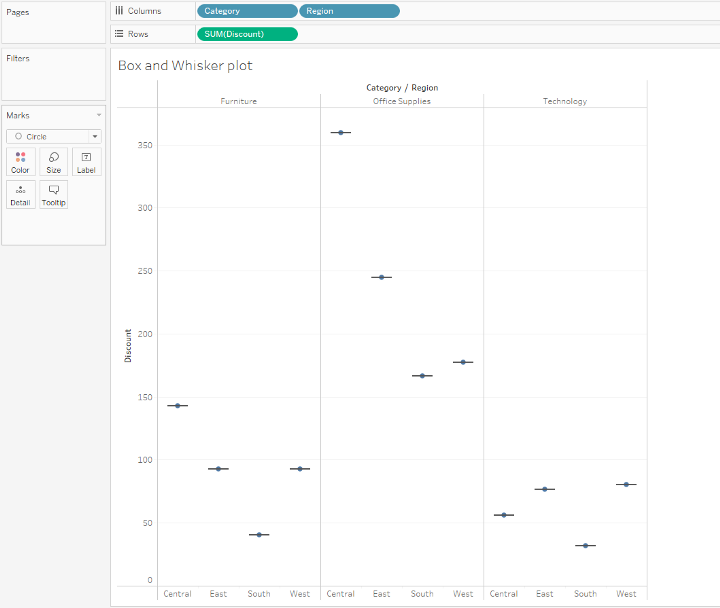top of pageSearch

# W ’s of Box and Whisker Plot

Let us discuss on (What, Why, When and How) a Box Plot.

A Box and Whisker Plot often called the Box plot is used to show the distribution of values along an axis. It is used to visualize five values in a dataset for the selected column(s) which is called the five number summary.

Let us understand the Five number summary in detail.

The Five number summary refers to the Minimum value, First Quartile (Q1), Median (Second Quartile Q2), Third quartile(Q3) and the Maximum value.Image by Author

The Median (50th percentile, Q2) is the value separating the higher half of a data sample from the lower half. In other words, it is the “middle” value of a data set.

First quartile (Q1/25th Percentile): The values between the smallest number and the median of the dataset.

Third quartile (Q3/75th Percentile): The values between the median and the highest value of the dataset.

Minimum value is calculated as Q1 -1.5*IQR

Maximum value is calculated as Q3 + 1.5*IQR

Inter-quartile range: The middle 50% of values fall within the inter-quartile range. Tableau draws a box around the interquartile range. The quartiles are called Hinge and Whiskers in Tableau.

Whiskers are the lines extending from the box on both the sides. They typically extend to 1.5*of the interquartile range to set boundary. Hence this plot is called the Box and whisker plot, the points beyond which would be considered Outliers.

When to use box plot

Box plot can be specifically used to find

• The key values such as the minimum, median, maximum, etc.

• Existence of outliers and their values

• Skewness and its direction

• If the data are symmetrical

• How tightly the data is grouped

How to make a Box plot

Let us make a Box plot that shows Discount by region and product Category.

1. Connect to Sample -Superstore data source

2. Drag the Category to columns and Discount to rowsImage by Author

Tableau creates a Bar chart by default

3.Now drag the Region to the columns, a bar chart as shown in the image is formed.Image by Author

4.Next Click show me in the tool bar and select the Box and Whisker plot type

5.Tableau displays a Box plot. We may notice that there are very few marks in each Box plot. Also, the regions from the column are shifted to the marks card.Image by Author

6.Drag the Regions back to the columns and we find that the horizontal lines are flattened, this is because the Box plot is based on a single mark and the data is aggregated in the current viewImage by Author

7.Now Disaggregate data by selecting Analysis> Aggregate measuresImage by Author

Instead of a single mark we can see a range of marks.Image by Author

8.Click the swap button to swap the axes,The Box plot now flows HorizontallyImage by Author

9.Right click the bottom axis and select the Edit Reference line where we can select an interesting color in the Fill drop down list.Image by Author

10.Box plot is now created with the selected color.Image by Author

By hovering across each plot, we can Interpret that there are outliers and the Discount provided to each Category across the Region.